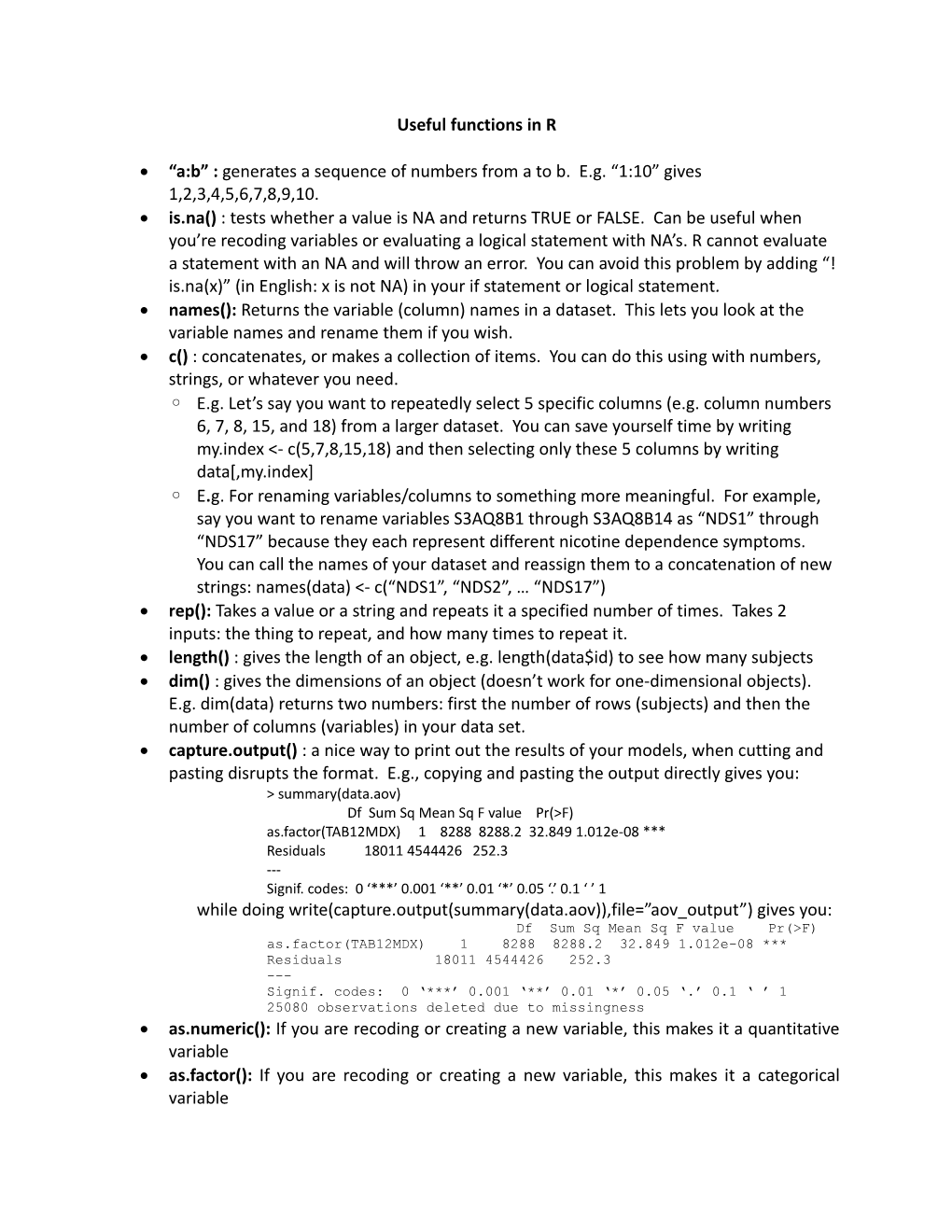# Useful Functions in RUseful functions in R

• “a:b” : generates a sequence of numbers from a to b. E.g. “1:10” gives 1,2,3,4,5,6,7,8,9,10.
• is.na() : tests whether a value is NA and returns TRUE or FALSE. Can be useful when you’re recoding variables or evaluating a logical statement with NA’s.R cannot evaluate a statement with an NA and will throw an error. You can avoid this problem by adding “!is.na(x)” (in English: x is not NA) in your if statement or logical statement.
• names(): Returns the variable (column) names in a dataset. This lets you look at the variable names and rename them if you wish.
• c() : concatenates, or makes a collection of items. You can do this using with numbers, strings, or whatever you need.

◦E.g. Let’s say you want to repeatedly select 5 specific columns (e.g. column numbers 6, 7, 8, 15, and 18) from a larger dataset. You can save yourself time by writing my.index <- c(5,7,8,15,18) and then selecting only these 5 columns by writing data[,my.index]

◦E.g. For renaming variables/columns to something more meaningful. For example, say you want to rename variables S3AQ8B1 through S3AQ8B14 as “NDS1” through “NDS17” because they each represent different nicotine dependence symptoms. You can call the names of your dataset and reassign them to a concatenation of new strings: names(data) <- c(“NDS1”, “NDS2”, … “NDS17”)

• rep(): Takes a value or a string and repeats it a specified number of times. Takes 2 inputs: the thing to repeat, and how many times to repeat it.
• length() : gives the length of an object, e.g. length(data\$id) to see how many subjects
• dim() : gives the dimensions of an object (doesn’t work for one-dimensional objects). E.g. dim(data) returns two numbers: first the number of rows (subjects) and then the number of columns (variables) in your data set.
• capture.output() : a nice way to print out the results of your models, when cutting and pasting disrupts the format. E.g., copying and pasting the output directly gives you:

> summary(data.aov)

Df Sum Sq Mean Sq F value Pr(>F)

as.factor(TAB12MDX) 1 8288 8288.2 32.849 1.012e-08 ***

Residuals 18011 4544426 252.3

---

Signif. codes: 0 ‘***’ 0.001 ‘**’ 0.01 ‘*’ 0.05 ‘.’ 0.1 ‘’ 1

while doing write(capture.output(summary(data.aov)),file=”aov_output”) gives you:

Df Sum Sq Mean Sq F value Pr(>F)

as.factor(TAB12MDX) 1 8288 8288.2 32.849 1.012e-08 ***

Residuals 18011 4544426 252.3

---

Signif. codes: 0 ‘***’ 0.001 ‘**’ 0.01 ‘*’ 0.05 ‘.’ 0.1 ‘ ’ 1

25080 observations deleted due to missingness

• as.numeric(): If you are recoding or creating a new variable, this makes it a quantitative variable
• as.factor(): If you are recoding or creating a new variable, this makes it a categorical variable
• mean(): Returns the mean/average of the input (usually a variable/column in dataset)
• sd(): Returns the standard deviation of the input (usually a variable/column in dataset)
• summary(): Returns a summary of the distribution of the input (or, returns a model summary if the input is a statistical model, e.g. a regression)